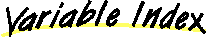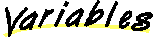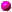`All Packages  Class Hierarchy  This Package  Previous  Next  Index`

# Class java.util.Vector

```java.lang.Object
|
+----java.util.Vector
```

public class Vector
extends Object
implements Cloneable, Serializable
The `Vector` class implements a growable array of objects. Like an array, it contains components that can be accessed using an integer index. However, the size of a `Vector` can grow or shrink as needed to accommodate adding and removing items after the `Vector` has been created.

Each vector tries to optimize storage management by maintaining a `capacity` and a `capacityIncrement`. The `capacity` is always at least as large as the vector size; it is usually larger because as components are added to the vector, the vector's storage increases in chunks the size of `capacityIncrement`. An application can increase the capacity of a vector before inserting a large number of components; this reduces the amount of incremental reallocation.

##capacityIncrement
The amount by which the capacity of the vector is automatically incremented when its size becomes greater than its capacity.elementCount
The number of valid components in the vector.elementData
The array buffer into which the components of the vector are stored.

##Vector()
Constructs an empty vector.Vector(int)
Constructs an empty vector with the specified initial capacity.Vector(int, int)
Constructs an empty vector with the specified initial capacity and capacity increment.

##addElement(Object)
Adds the specified component to the end of this vector, increasing its size by one.capacity()
Returns the current capacity of this vector.clone()
Returns a clone of this vector.contains(Object)
Tests if the specified object is a component in this vector.copyInto(Object[])
Copies the components of this vector into the specified array.elementAt(int)
Returns the component at the specified index.elements()
Returns an enumeration of the components of this vector.ensureCapacity(int)
Increases the capacity of this vector, if necessary, to ensure that it can hold at least the number of components specified by the minimum capacity argument.firstElement()
Returns the first component of this vector.indexOf(Object)
Searches for the first occurence of the given argument, testing for equality using the `equals` method.indexOf(Object, int)
Searches for the first occurence of the given argument, beginning the search at `index`, and testing for equality using the `equals` method.insertElementAt(Object, int)
Inserts the specified object as a component in this vector at the specified `index`.isEmpty()
Tests if this vector has no components.lastElement()
Returns the last component of the vector.lastIndexOf(Object)
Returns the index of the last occurrence of the specified object in this vector.lastIndexOf(Object, int)
Searches backwards for the specified object, starting from the specified index, and returns an index to it.removeAllElements()
Removes all components from this vector and sets its size to zero.removeElement(Object)
Removes the first occurrence of the argument from this vector.removeElementAt(int)
Deletes the component at the specified index.setElementAt(Object, int)
Sets the component at the specified `index` of this vector to be the specified object.setSize(int)
Sets the size of this vector.size()
Returns the number of components in this vector.toString()
Returns a string representation of this vector.trimToSize()
Trims the capacity of this vector to be the vector's current size.

##elementData
``` protected Object elementData[]
```
The array buffer into which the components of the vector are stored. The capacity of the vector is the length of this array buffer.elementCount
``` protected int elementCount
```
The number of valid components in the vector.capacityIncrement
``` protected int capacityIncrement
```
The amount by which the capacity of the vector is automatically incremented when its size becomes greater than its capacity. If the capacity increment is `0`, the capacity of the vector is doubled each time it needs to grow.

##Vector
``` public Vector(int initialCapacity,
int capacityIncrement)
```
Constructs an empty vector with the specified initial capacity and capacity increment.

Parameters:
initialCapacity - the initial capacity of the vector.
capacityIncrement - the amount by which the capacity is increased when the vector overflows.Vector
``` public Vector(int initialCapacity)
```
Constructs an empty vector with the specified initial capacity.

Parameters:
initialCapacity - the initial capacity of the vector.Vector
``` public Vector()
```
Constructs an empty vector.

##copyInto
``` public final synchronized void copyInto(Object anArray[])
```
Copies the components of this vector into the specified array. The array must be big enough to hold all the objects in this vector.

Parameters:
anArray - the array into which the components get copied.trimToSize
``` public final synchronized void trimToSize()
```
Trims the capacity of this vector to be the vector's current size. An application can use this operation to minimize the storage of a vector.ensureCapacity
``` public final synchronized void ensureCapacity(int minCapacity)
```
Increases the capacity of this vector, if necessary, to ensure that it can hold at least the number of components specified by the minimum capacity argument.

Parameters:
minCapacity - the desired minimum capacity.setSize
``` public final synchronized void setSize(int newSize)
```
Sets the size of this vector. If the new size is greater than the current size, new `null` items are added to the end of the vector. If the new size is less than the current size, all components at index `newSize` and greater are discarded.

Parameters:
newSize - the new size of this vector.capacity
``` public final int capacity()
```
Returns the current capacity of this vector.

Returns:
the current capacity of this vector.size
``` public final int size()
```
Returns the number of components in this vector.

Returns:
the number of components in this vector.isEmpty
``` public final boolean isEmpty()
```
Tests if this vector has no components.

Returns:
`true` if this vector has no components; `false` otherwise.elements
``` public final synchronized Enumeration elements()
```
Returns an enumeration of the components of this vector.

Returns:
an enumeration of the components of this vector.
Enumerationcontains
``` public final boolean contains(Object elem)
```
Tests if the specified object is a component in this vector.

Parameters:
elem - an object.
Returns:
`true` if the specified object is a component in this vector; `false` otherwise.indexOf
``` public final int indexOf(Object elem)
```
Searches for the first occurence of the given argument, testing for equality using the `equals` method.

Parameters:
elem - an object.
Returns:
the index of the first occurrence of the argument in this vector; returns `-1` if the object is not found.
equalsindexOf
``` public final synchronized int indexOf(Object elem,
int index)
```
Searches for the first occurence of the given argument, beginning the search at `index`, and testing for equality using the `equals` method.

Parameters:
elem - an object.
index - the index to start searching from.
Returns:
the index of the first occurrence of the object argument in this vector at position `index` or later in the vector; returns `-1` if the object is not found.
equalslastIndexOf
``` public final int lastIndexOf(Object elem)
```
Returns the index of the last occurrence of the specified object in this vector.

Parameters:
elem - the desired component.
Returns:
the index of the last occurrence of the specified object in this vector; returns `-1` if the object is not found.lastIndexOf
``` public final synchronized int lastIndexOf(Object elem,
int index)
```
Searches backwards for the specified object, starting from the specified index, and returns an index to it.

Parameters:
elem - the desired component.
index - the index to start searching from.
Returns:
the index of the last occurrence of the specified object in this vector at position less than `index` in the vector; `-1` if the object is not found.elementAt
``` public final synchronized Object elementAt(int index)
```
Returns the component at the specified index.

Parameters:
index - an index into this vector.
Returns:
the component at the specified index.
Throws: ArrayIndexOutOfBoundsException
if an invalid index was given.firstElement
``` public final synchronized Object firstElement()
```
Returns the first component of this vector.

Returns:
the first component of this vector.
Throws: NoSuchElementException
if this vector has no components.lastElement
``` public final synchronized Object lastElement()
```
Returns the last component of the vector.

Returns:
the last component of the vector, i.e., the component at index `size() - 1`.
Throws: NoSuchElementException
if this vector is empty.setElementAt
``` public final synchronized void setElementAt(Object obj,
int index)
```
Sets the component at the specified `index` of this vector to be the specified object. The previous component at that position is discarded.

The index must be a value greater than or equal to `0` and less than the current size of the vector.

Parameters:
obj - what the component is to be set to.
index - the specified index.
Throws: ArrayIndexOutOfBoundsException
if the index was invalid.
sizeremoveElementAt
``` public final synchronized void removeElementAt(int index)
```
Deletes the component at the specified index. Each component in this vector with an index greater or equal to the specified `index` is shifted downward to have an index one smaller than the value it had previously.

The index must be a value greater than or equal to `0` and less than the current size of the vector.

Parameters:
index - the index of the object to remove.
Throws: ArrayIndexOutOfBoundsException
if the index was invalid.
sizeinsertElementAt
``` public final synchronized void insertElementAt(Object obj,
int index)
```
Inserts the specified object as a component in this vector at the specified `index`. Each component in this vector with an index greater or equal to the specified `index` is shifted upward to have an index one greater than the value it had previously.

The index must be a value greater than or equal to `0` and less than or equal to the current size of the vector.

Parameters:
obj - the component to insert.
index - where to insert the new component.
Throws: ArrayIndexOutOfBoundsException
if the index was invalid.
sizeaddElement
``` public final synchronized void addElement(Object obj)
```
Adds the specified component to the end of this vector, increasing its size by one. The capacity of this vector is increased if its size becomes greater than its capacity.

Parameters:
obj - the component to be added.removeElement
``` public final synchronized boolean removeElement(Object obj)
```
Removes the first occurrence of the argument from this vector. If the object is found in this vector, each component in the vector with an index greater or equal to the object's index is shifted downward to have an index one smaller than the value it had previously.

Parameters:
obj - the component to be removed.
Returns:
`true` if the argument was a component of this vector; `false` otherwise.removeAllElements
``` public final synchronized void removeAllElements()
```
Removes all components from this vector and sets its size to zero.clone
``` public synchronized Object clone()
```
Returns a clone of this vector.

Returns:
a clone of this vector.
Overrides:
clone in class ObjecttoString
``` public final synchronized String toString()
```
Returns a string representation of this vector.

Returns:
a string representation of this vector.
Overrides:
toString in class Object

`All Packages  Class Hierarchy  This Package  Previous  Next  Index`

Submit a bug or feature - Version 1.1.8 of Java Platform API Specification
Java is a trademark or registered trademark of Sun Microsystems, Inc. in the US and other countries.